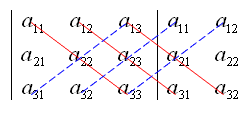## 定义

### 直观定义

$\det(A)=\sum _{\sigma \in S_{n}}\operatorname {sgn}(\sigma )\prod _{i=1}^{n}a_{i,\sigma (i)}$

• $S_n$: 集合 $\left\{1,2,...,n\right\}$ 上置换的全体，即集合 $\left\{1,2,...,n\right\}$ 到自身上的一一映射（双射）的全体，一共有 $A_{nn} = n!$ 个元素，，因此上式中共有 $n!$ 个求和项。$S_n$ 中每个元素 $\sigma$ 都是一个映射关系，给定一个输入 $i$，给出一个输出。

• $\operatorname {sgn}(\sigma )$ 表示置换 $\sigma \in S_{n}$符号差，具体地说，满足 $1\leq i\leq j\leq n$$\sigma (i)>\sigma (j)$ 的有序数对 $\left(i,j\right)$ 称为 $\sigma$ 的一个逆序。如果 $\sigma$ 的逆序共有偶数个，则 $\operatorname {sgn} \sigma =1$，如果共有奇数个，则 $\operatorname {sgn} \sigma =-1$

举例来说，对于 3 元置换 $\sigma =\left(2,3,1\right)$（即是说 $\sigma (1)=2}，\sigma (2)=3}， \sigma (3)=1$）而言，由于 1 在 2 后，1 在 3 后，所以共有 2 个逆序（偶数个），因此 $\operatorname {sgn} (\sigma )=1$，从而 3 阶行列式中项 $a_{1,2}a_{2,3}a_{3,1}$ 的符号是正的。

• $a_{ij}$: 矩阵 $A$ 的第 $i$ 行第 $j$ 列的元素

${\begin{vmatrix}a_{1,1}&a_{1,2}\\a_{2,1}&a_{2,2}\end{vmatrix}}=a_{1,1}a_{2,2}-a_{1,2}a_{2,1}}$$\displaystyle {\begin{vmatrix}a_{1,1}&a_{1,2}&a_{1,3}\\a_{2,1}&a_{2,2}&a_{2,3}\\a_{3,1}&a_{3,2}&a_{3,3}\end{vmatrix}} \\ =a_{1,1}a_{2,2}a_{3,3}+a_{1,2}a_{2,3}a_{3,1}+a_{1,3}a_{2,1}a_{3,2} \\ \quad -a_{1,3}a_{2,2}a_{3,1}-a_{1,1}a_{2,3}a_{3,2}-a_{1,2}a_{2,1}a_{3,3}$

### 拉普拉斯展开（代数余子式展开法）

$M_{ij}={\begin{vmatrix}m_{1,1}&\dots &m_{1,j-1}&m_{1,j+1}&\dots &m_{1,n}\\\vdots &&\vdots &\vdots &&\vdots \\m_{i-1,1}&\dots &m_{i-1,j-1}&m_{i-1,j+1}&\dots &m_{i-1,n}\\m_{i+1,1}&\dots &m_{i+1,j-1}&m_{i+1,j+1}&\dots &m_{i+1,n}\\\vdots &&\vdots &\vdots &&\vdots \\m_{n,1}&\dots &m_{n,j-1}&m_{n,j+1}&\dots &m_{n,n}\end{vmatrix}}$

$M$ 关于元素 $m_{ij}$ 的代数余子式记作 $C_{ij}$$C_{ij}=(-1)^{(i+j)}\cdot M_{ij}$

$\det {M}=\sum _{j=1}^{n}m_{ij}C_{i,j}, 按第 i 行展开 \\ det {M}=\sum _{i=1}^{n}m_{ij}C_{i,j}, 按第 j 列展开 \\$

{\begin{aligned}|A|={\begin{vmatrix}a&b\\c&d\end{vmatrix}}=ad-bc.\end{aligned}}}

{\begin{aligned}|A|={\begin{vmatrix}a&b&c\\d&e&f\\g&h&i\end{vmatrix}}&=a\,{\begin{vmatrix}\Box &\Box &\Box \\\Box &e&f\\\Box &h&i\end{vmatrix}}-b\,{\begin{vmatrix}\Box &\Box &\Box \\d&\Box &f\\g&\Box &i\end{vmatrix}}+c\,{\begin{vmatrix}\Box &\Box &\Box \\d&e&\Box \\g&h&\Box \end{vmatrix}}\\[3pt]&=a\,{\begin{vmatrix}e&f\\h&i\end{vmatrix}}-b\,{\begin{vmatrix}d&f\\g&i\end{vmatrix}}+c\,{\begin{vmatrix}d&e\\g&h\end{vmatrix}}\\[3pt]&=aei+bfg+cdh-ceg-bdi-afh.\end{aligned}}}

${\begin{vmatrix}a&b&c&d\\e&f&g&h\\i&j&k&l\\m&n&o&p\end{vmatrix}}=a\,{\begin{vmatrix}f&g&h\\j&k&l\\n&o&p\end{vmatrix}}-b\,{\begin{vmatrix}e&g&h\\i&k&l\\m&o&p\end{vmatrix}}+c\,{\begin{vmatrix}e&f&h\\i&j&l\\m&n&p\end{vmatrix}}-d\,{\begin{vmatrix}e&f&g\\i&j&k\\m&n&o\end{vmatrix}}.}$

\begin{aligned} \det(M) &=\sum _{\sigma \in S_{n}}\operatorname {sgn}(\sigma )\prod _{i=1}^{n}m_{i,\sigma (i)} \end{aligned}

$\sum _{\sigma \in S_{n}}\operatorname {sgn}(\sigma )\prod _{i=1}^{n}m_{i,\sigma (i)} = \sum_{j=1}^nm_{ij}C_{ij} = \sum_{j=1}^n(-1)^{i+j}m_{ij}M_{ij}$

\begin{aligned} & \operatorname{sgn} ({\tau}) \cdot m_{1, \tau(1)} \cdots m_{i, j}, \cdots m_{n, \tau(n)} \\ &= \operatorname{sgn}(\tau) \cdot m_{ij} \cdot m_{1, \tau(1)} \cdots m_{i-1, \tau(i-1)}m_{i+1, \tau(i+1)} \cdots m_{n, \tau(n)} \\ &\xlongequal{记 M_{ij} 的元素为 a_{st}} \operatorname{sgn}(\tau) \cdot m_{ij} \cdot a_{1, \sigma(1)} \cdots a_{i-1, \sigma(i-1)}a_{i, \sigma(i)} \cdots a_{n-1, \sigma(n-1)} \\ \end{aligned}

$\tau \,=(n,n-1,\ldots ,i)\,\sigma '\,(j,j+1,\ldots ,n)}。$

$\begin{pmatrix} n & n-1 & n-2 & \cdots & i+1 & i \\ n-1 & n-2 & n-3 & \cdots & i & n \\ \sigma'(n-1) & \sigma'(n-2) & \sigma'(n-3) & \cdots & \sigma'(i) & n \\ \begin{cases} \sigma'(n-1), \sigma'(n-1) < j \\ \sigma'(n-1)+1, \sigma'(n-1) \ge j \\ \end{cases} & \begin{cases} \sigma'(n-2), \sigma'(n-2) < j \\ \sigma'(n-2)+1, \sigma'(n-2) \ge j \\ \end{cases} & \begin{cases} \sigma'(n-3), \sigma'(n-3) < j \\ \sigma'(n-3)+1, \sigma'(n-3) \ge j \\ \end{cases} & \cdots & \begin{cases} \sigma'(i), \sigma'(i) < j \\ \sigma'(i)+1, \sigma'(i) \ge j \\ \end{cases} & j \end{pmatrix}$

$\operatorname {sgn} (\tau) \,=(-1)^{2n-(i+j)}\operatorname {sgn} (\sigma) '\,=(-1)^{i+j}\operatorname {sgn} (\sigma) }$

\begin{aligned} \sum _{\tau \in S_{n}, \tau(i) = j}\operatorname {sgn}(\tau )\prod _{i=1}^{n}m_{i,\tau (i)} &= \sum_{\sigma \in S_{n-1}} (-1)^{i+j}\operatorname {sgn} \sigma \cdot m_{ij} \cdot a_{i, \sigma(1)} \cdots a_{n-1, \tau(n-1)} \\ &= (-1)^{i+j} m_{ij}M_{ij} \end{aligned}

$\det(A) = \sum_{j=1}^n \left( \sum _{\tau \in S_{n}, \tau(i) = j}\operatorname {sgn}(\tau )\prod _{i=1}^{n}m_{i,\tau (i)} \right) = \sum_{j=1}^n (-1)^{i+j} m_{ij}M_{ij} = \sum_{j=1}^n m_{ij}C_{ij}$

TODO

TODO

TODO

TODO